# Problem of the day! (Algebra)

in #ocd8 months ago

Man in black started walking from point O at uniform speed of 3.5kph. After 3 hours, Man in blue started walking from the same point and walked 3.5km in first hour, 4km in the second hour and so on, increasing 0.5km per hour. How many hours after the Man in black left point O will he be caught by Man in blue?

Solution:

Man in black started walking from point O at uniform speed of 3.5kph.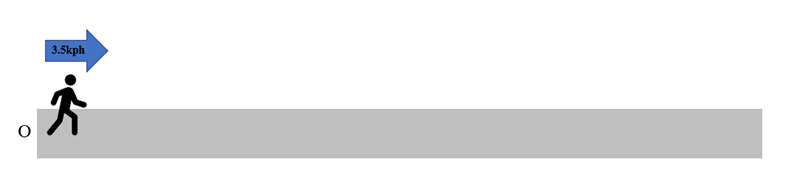After 3 hours;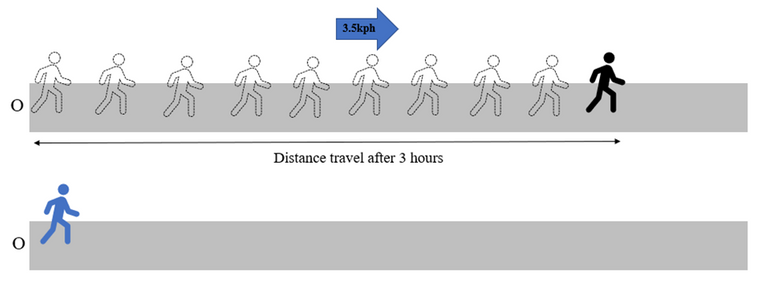After 1 hour;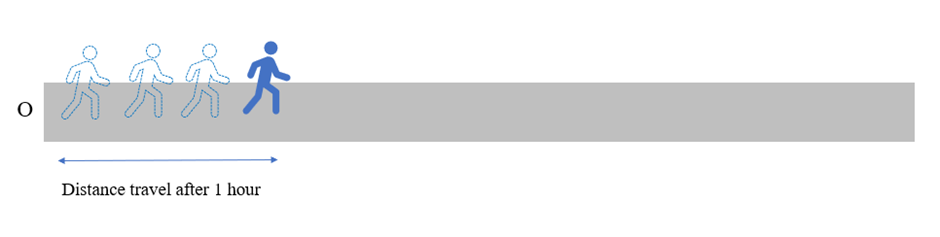Man in Blue’s distance increasing 0.5km per hour.

Therefore, in order to caught the Man in black their distances must be equal.

So,

Distance of Man in black = Distance of Man in blue

Distance of Man in black = 3.5(n+3)
Distance in Man in blue = n/2(a1 +an)
where;
a1 = 3.5
an = a1 + (n-1)d
an = 3.5+(n-1)d
and d = 0.5km per hour
so,
3.5(n+3) = (n/2)[3.5 + 3.5+(n-1)(.5)]
3.5(n+3) = (n/2)(6.5 + 0.5n)
7(n+3) = 6.5n+0.5n^2
7n + 21 = 6.5n +0.5n^2
0.5n^2 -0.5n-21=0
solve for n using quadratic formula
n = [-b±(b^2 -4ac)^(0.5)]/2a
where
a = 0.5
b = -0.5
c = -21
n = {-(-0.5)±[(0.5)^2 -4(0.5)(-21)]^(0.5)}/2(0.5)
n = -6 and 7
use n = 7
How many hours after the Man in black left point O will he be caught by Man in blue?
Time the man in black get caught by man in blue = n+3
Time the man in black get caught by man in blue = 7+3 =10 hours (answer)

Sort:

Congratulations @exilldiogolvin! You have completed the following achievement on the Hive blockchain and have been rewarded with new badge(s):You published more than 10 posts.Your next target is to reach 20 posts.

If you no longer want to receive notifications, reply to this comment with the word `STOP`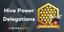Our Hive Power Delegations to the June PUM Winners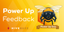Feedback from the July 1st Hive Power Up Day